# Test: Fixed Bias & Emitter Bias

## 10 Questions MCQ Test Analog Circuits | Test: Fixed Bias & Emitter Bias

Description
Attempt Test: Fixed Bias & Emitter Bias | 10 questions in 10 minutes | Mock test for Electronics and Communication Engineering (ECE) preparation | Free important questions MCQ to study Analog Circuits for Electronics and Communication Engineering (ECE) Exam | Download free PDF with solutions
QUESTION: 1

### Whichof the following is the correct relatioship between IB and IE?

Solution:

For a BJT, the collector and emitter current are related as IE = (β + 1) IB.

QUESTION: 2

### For best operation of a BJT, which region must the operating point be set at?

Solution:

Operating point for a BJT must always be set in the active region to ensure proper functioning. Setting up of Q-point in any other region may lead to reduced functionality.

QUESTION: 3

### From the given circuit, what is the value of IBQ?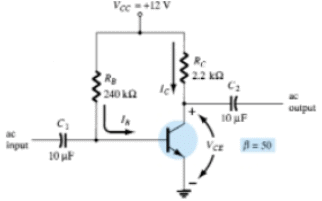Solution:

We know that IBQ = (VCC – VBE)/RB = 11.3/240 uA = 47.08 uA.

QUESTION: 4

From the given circuit, what is the value of VCEQ?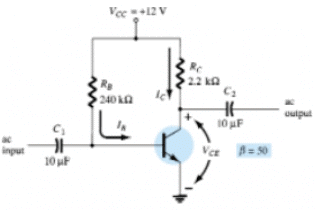Solution:

VCEQ = VCC – ICRC. Hence, VCEQ = 6.83 V.

QUESTION: 5

From the given circuit, what is the value of VBC?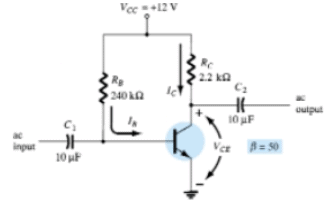Solution:

VBC = VB – VC = VBE – VCE = -6.13 V.

QUESTION: 6

From the given circuit, what is the value of the saturation collector current?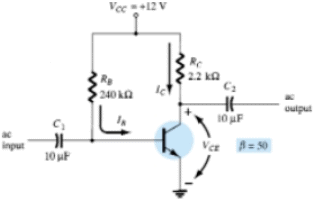Solution:

Saturation collector current = VCC/RC = 5.45 mA.

QUESTION: 7

In the given circuit, what is the value of IC?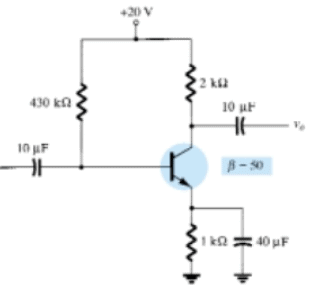Solution:

β IB = 2.01 mA.

QUESTION: 8

In the given circuit, what is the value of VCE?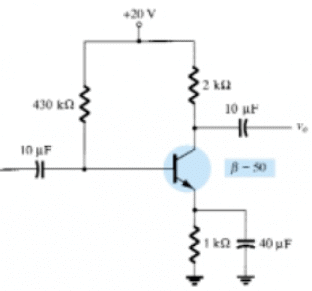Solution:

VCE = VCC – ICRC = 13.97 V.

QUESTION: 9

In the given circuit, what is the value of VE?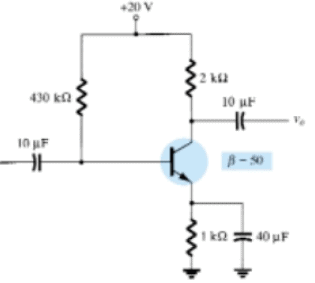Solution:

VE = VC – VCE = 2.01 V.

QUESTION: 10

In the given circuit, what is the value of saturation collector current?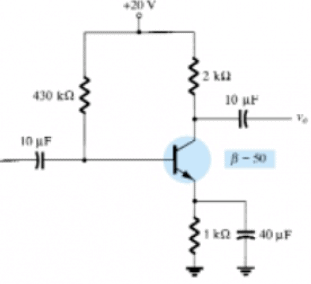Solution:

Saturation collector current = VCC/(RC + RE) = 6.67 mA.Use Code STAYHOME200 and get INR 200 additional OFF Use Coupon Code